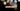Leetcode | Solution of Two Sum II in JavaScript

September 30th, 2019
|

In this post, we will solve two sum II - input array is sorted problem from leetcode. Let's begin.

Problem Statement

The question can be found at Leetcode Two Sum II - Input array is sorted.

The problem states that we are given an array of integers and a target number, our task is to return the indices of the two numbers which adds up to the target.

Constraints and challenges

• Input array is sorted in ascending order.

Solution

We have already discussed the solution of two sum problem in multiple ways in my earlier blog. In this case, the array is sorted which makes it easier to find the solution. I will list down one of the solutions for the problem, for the rest please refer to my two sum blog post.

Two pointer method

Two pointer ( finger ) method is a very famous method of solving problems where you take two elements on opposite ends of the array and move inwards, in turn covering all elements.

Let's take an example.

In the following array

let arr = [1,2,3,4,5,6,7,8]

Notice the sorted array.

If we were given a target and had to find the indices of numbers, instead of iterating on one end, we can have two pointers, one at index 0 and another at the end.

Take the sum, if the sum is more than the target, we need to decrease the sum. Well if we move the left pointer to the next right position, it will take us to a number larger than the earlier one, because of the sorted array. So we move our right pointer to next left position, thus decreasing the sum.

Similarly, if the sum is less than the target, we need to increase the sum. Well if we move the right pointer to the next left position, it will take us to a number smaller than the earlier one. So we move our left pointer to next right position, thus increasing the sum.

If the sum is equal to the target, we got our result. All good. If lower pointer (left one) becomes equal to right one, we stop because we have already covered all elements and no solution found.

var twoSum = function (numbers, target) {
let i = 0;
let j = numbers.length - 1;
while (i !== j) {
if (numbers[i] + numbers[j] > target) {
j--;
} else if (numbers[i] + numbers[j] < target) {
i++;
} else {
return [i + 1, j + 1];
}
}
};

Stats

Status: Accepted
Runtime: 52ms
Memory: 35.2MB

Time and space complexity

Space complexity

Well, we have an array as input and a number, a couple of variables to store left and right pointers. So space complexity is O(n)

Time Complexity

In the worst case we will loop over the array once, so time complexity will be O(n)

Summary

A very quick blog didn't want to stretch this a lot because had already done two sum extensively before. Please refer to two sum blog for more details.

I hope you enjoyed solving this question. This is it for this one, complete source code for this post can be found on my Github Repo. Will see you in the next one.